New update is available. Click here to update.Close
Topic list
Ninja and Tree
NINJA
Trees
Topics (Covered in this problem)
Problem solved
Skill meterTrees
-
-
Other topics
Problem solved
Skill meterStrings
-
-Matrices (2D Arrays)
-
--
-Sorting
-
-Binary Search
-
-Stacks & Queues
-
-Graph
-
-Dynamic Programming
-
-Greedy
-
-Tries
-
-Arrays
-
-SQL
-
-Binary Search Trees
-
-Heap
-
-Bit Manipulation
-
-
Solve problems & track your progress
Checkout your overall progress in every topic here
BecomeSensei
in DSA topics
Open the topic and solve more problems associated with it to improve your skills
Check out the skill meter for every topic
See how many problems you are left with to solve for cracking any stage. Score more than zero to get your progress counted.

# Ninja and Tree

Ninja0/200Share0 upvotes

## Problem Statement

#### 2) Find the number of different colours in the subtree of the vertex ‘QUERIES[i]’.

##### For Example:
``````Let ‘N’ = 5, ‘EDGES’ = [[1, 2], [1, 3], [2, 4], [2, 5]], ‘QUERIES’ = [[1, 2, 3], [2, 1], [1, 3, 2], [2, 1]] and ‘COLOR’ = [1, 1, 1, 1, 1].
The initial tree will be:
``````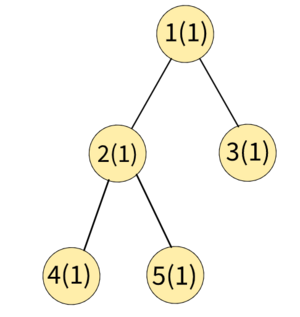``````After processing the query [1, 2, 3] i.e. colour all vertices in the subtree of 2 to 3.
``````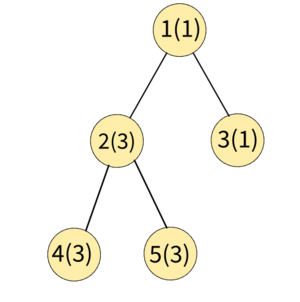``````Now the number of different colours in subtree of the vertex 1 is 2.
After processing the query [1, 3, 2] i.e. colour all vertices in the subtree of 3 to 2.
``````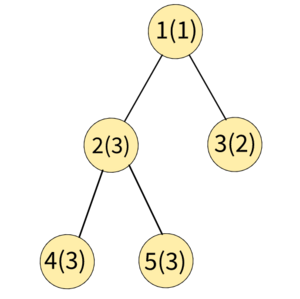``````Now the number of different colours in subtree of the vertex 1 are 3.
Hence the answer to this test case is 2 and 3 (print on separate lines).
``````
Detailed explanation ( Input/output format, Notes, Constraints, Images )##### Sample Input 1 :
``````2
5 4
1 1 1 1 1
1 2
1 3
2 4
2 5
1 2 3
2 1
1 3 2
2 1
3 2
1 1 1
1 2
1 3
1 2 2
2 1
``````
##### Sample Output 1 :
``````2
3
2
``````
##### Explanation of Sample Output 1 :
``````Test Case 1:
The initial tree will be:
````````````After processing the query [1, 2, 3] i.e. color all vertices in subtree of 2 to 3.
````````````Now the number of different colors in subtree of the vertex 1 are 2.
After processing the query [1, 3, 2] i.e. color all vertices in subtree of 3 to 2.
````````````Now the number of different colors in subtree of the vertex 1 are 3.
Hence the answer to this test case is 2 and 3 (print on separate lines).

Test Case 2:
The initial tree will be:
``````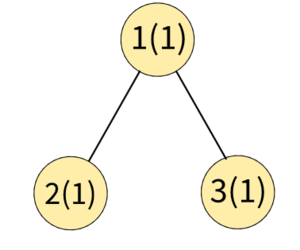``````After processing the query [1, 2, 2] i.e. color all vertices in subtree of 2 to 2.
``````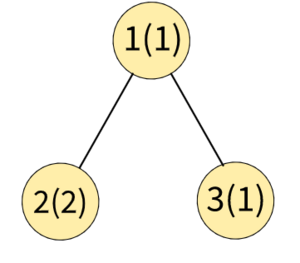``````Now the number of different colors in the subtree of the vertex 1 are 2.
Hence the answer to this test case is 2.
``````
##### Sample Input 2 :
``````2
2 2
1 3
1 2
1 1 2
2 1
1 1
1
2 1
``````
##### Sample Output 2 :
``````1
1
``````
##### Explanation of Sample Output 2 :
``````Test Case 1:
The initial tree will be:
``````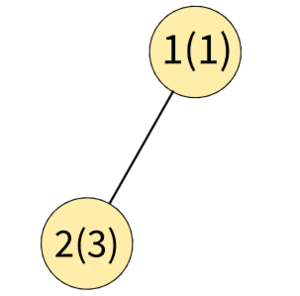``````After processing the query [1, 1, 2] i.e. color all vertices in the subtree of 1 to 2.
``````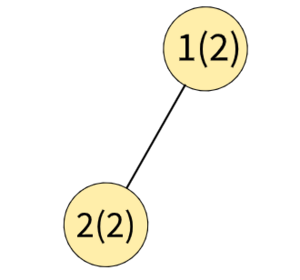``````Now the number of different colors in subtree of vertex 1 is 1. Hence answer to this testcase will be 1.

Test Case 2:
Initially, tree will be:
````````````The number of different colors in the subtree of vertex 1 is only 1. Hence answer to this testcase will be 1.
``````AutoConsole